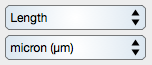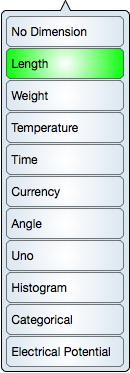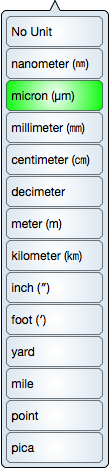Graph IDEControls ► Unit Selector

A Unit Selector is a combination of two pop up buttons, as shown in the following diagram, along with their respective menus.First, set a dimension using the top pop up button and then set a unit that is appropriate for that dimension using the lower pop up button.

The Unit Selector appears on many of the inspector editors, for example the Single Coordinate Graph and the spreadsheet. A Unit Selector without specifying dimension is incorporated into the Graphic View editor. In that case, the dimension menu is not required because the dimension is always length.

The unit type is not enough to specify a unit and the dimension is also required. For example, ounce is both a weight and volume. In Graph IDE (particularly its coding) the dimension and unit type are referred to as Quantity Type and the value with a particular unit is referred to as a Quantity Value. The type and value together are referred to as Quantity. There is another concept called Depiction which is the way the Quantity is represented. See Color Selector for an example of that.

Dimension SelectorUse the dimension selector (pop up button menu) to select a dimension. This diagram shows length as the selected dimension type. Once the dimension is selected then the unit selector is populated with values appropriate to that dimension.

See the table below for a description of the types.

 No Dimension Specifies that the corresponding value is unitless. Length The length dimension includes metric, imperial and typographic units Weight The weight dimension includes metric, imperial, and atomic mass units. Temperature The temperature dimension includes metric, imperial and physics (kelvin) units. Time The time dimension includes imperial and calendar units. Note that that time is split between uniform and non-uniform units. For example, each second is the same span of time whereas a month can be a span of time from 28 to 31 days in the Gregorian calendar and a different amount of days in a different calendar system. The unit types do not imply the calendar type. Currency The currency dimension includes many international currencies. Currency is a dimension with units but the scale between units varies constantly (via exchange rates) making this dimension particularly interesting. In many systems Currency is not thought of as a fundamental dimension. Angle The angle dimension is either (imperial) degrees or radians. By convention, all angle units, such as rotation, are specified in degrees. Uno The uno dimension specifies units as decimal increments of the value one. For example, percent, parts per decimal base, etc. This is particularly needed when a value is expressed in percentages. Byte The byte dimension specifies units as bytes, both 2 (e.g. kilo) and 10 (e.g. kibi) base. Histogram The histogram dimension is reserved for histograms only. Typically, this is not considered a dimension but because histograms are available as a graph then it is convenient to give the graph dimension a unique unit. Categorical The Categorical dimension specifies some discrete dimension types. This is useful for bar charts, but is also useful for counting controls such as a table column or row specifier where there are not fractional amounts. Electrical Potential The electrical potential dimension specifies several metric volt units. Note that this dimension is usually synonymous with its unit because electrical potential is only specified in volts and there is no other unit type.

Unit Selector

Use the unit selector (pop up button menu) to select a unit. This diagram shows possible length units with the micron unit selected. If the unit has a symbol then that appears in parenthesis after the unit name.Units are a standard concept and are for the most part self explanatory. However, the categorical dimension and corresponding units may require a bit of explanation as given in the table below.

The categorical dimension is not really a dimension per se and its units are really set-theoretic concepts. However, it is incorporated into the unit controls because many controls and graphs have values that are thought of in terms of a discrete variable all homomorphic to counting numbers. Note that this dimension is not named "discrete" because all other dimensions can have a discrete quality (for example, currency can be in basis points).

 Nominal This is the typical categorical type in statistics where the variables do not have an order. Note that in some statistical circles, Nominal is simply referred to as Categorical and Ordinal is a subtype of Categorical. Ordinal This is the typical categorical type in statistics where the variables have an order. Although this is simply conceptual, many dimensions are ordinal in the sense that they have a metric operation for ordering. Dichotomous Dichotomous is the binary unit of yes or no. It can also be referred to in many depictions such as the word "choice" or "on" and "off". Note the strong distinction between unit and depiction. Integer Integer is all non-fractional numbers. This is a set theoretic concept and not necessarily a unit. Whole Number All positive integers including zero are whole numbers. The worth of a unit is in its applicability and the whole number unit is utilized in the Color Map count and is particularly comforting to realize that the value can be zero (ℕ₀). Natural Number All positive integers (excluding zero) are defined as natural numbers in this implementation. The worth of a unit is in its applicability and the natural number unit is utilized in the Table controls to specifically indicate that the row and column indices begin at one (and not zero). A Natural Number may also be referred to as a counting number and sometimes a natural number includes zero, but not in this implementation. As such the set theoretic double-struck N is subscripted with a one to make it explicit that natural numbers begin at one (ℕ₁).

What makes a dimension and the set of units within that dimension clear is the fact that each unit [value] can be mapped into another unit [value] without a model specifying a different dimension (unit analysis). This is no clearer than with the Categorical dimension where the relationship between units within the categorical dimension is a simple matter of perception rather than transformation. For example, the ordinal unit is often mapped to integers as a matter of convenience to make the sequence index of the categorical value explicit. This is mere trickery as it is purely conceptual.